# Selina Solutions Concise Maths Class 7 Chapter 13: Set Concepts Exercise 13D

Selina Solutions Concise Maths Class 7 Chapter 13 Set Concepts Exercise 13D has answers curated by knowledgeable faculty at BYJU’S, which are 100% accurate. The solutions purely follow the ICSE syllabus to help students perform well in the annual exam. The union, intersection and difference of sets is the major concept discussed under this exercise. Students can refer to the solutions PDF to understand the various methods of solving problems effortlessly. Selina Solutions Concise Maths Class 7 Chapter 13 Set Concepts Exercise 13D, PDF can be downloaded from the links which are available below.

## Selina Solutions Concise Maths Class 7 Chapter 13: Set Concepts Exercise 13D Download PDF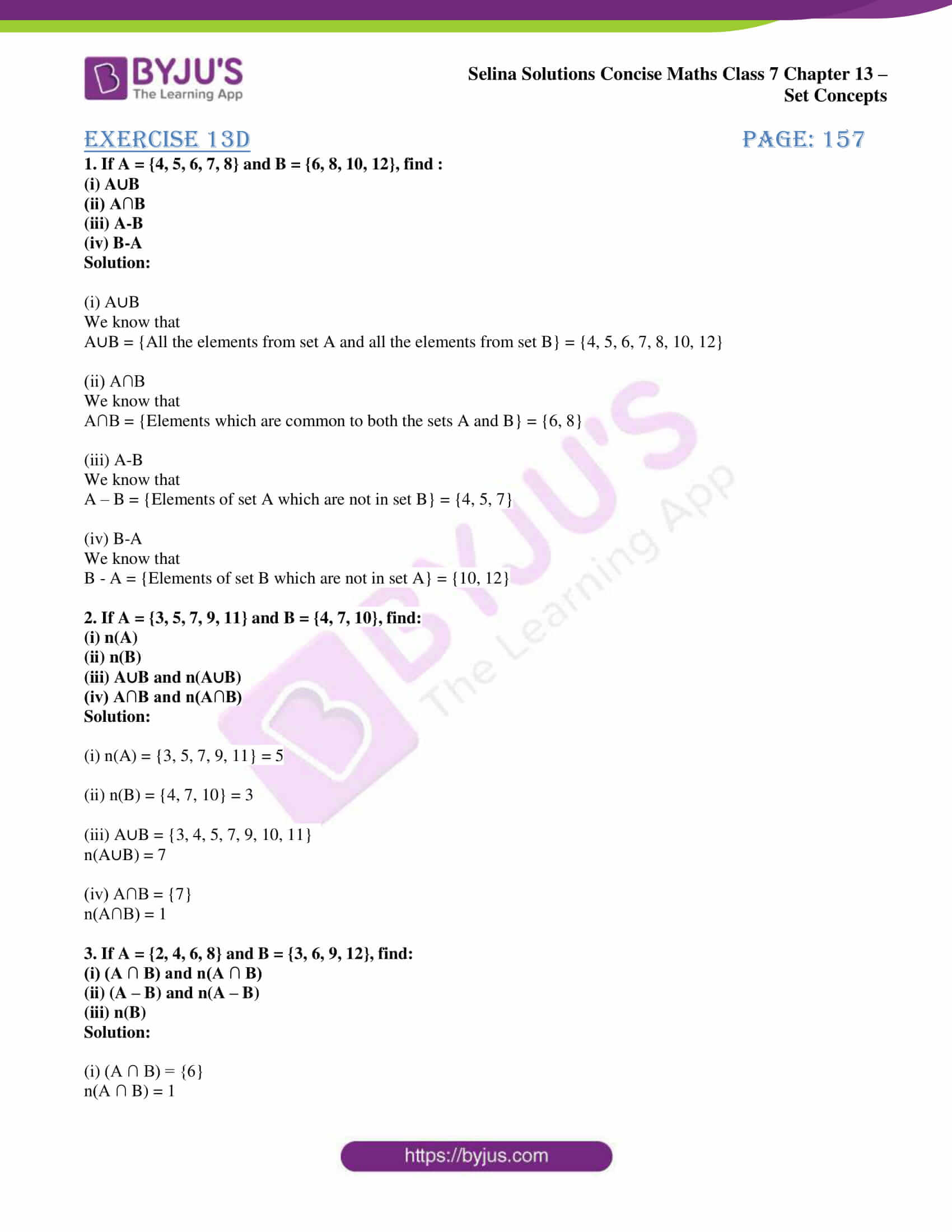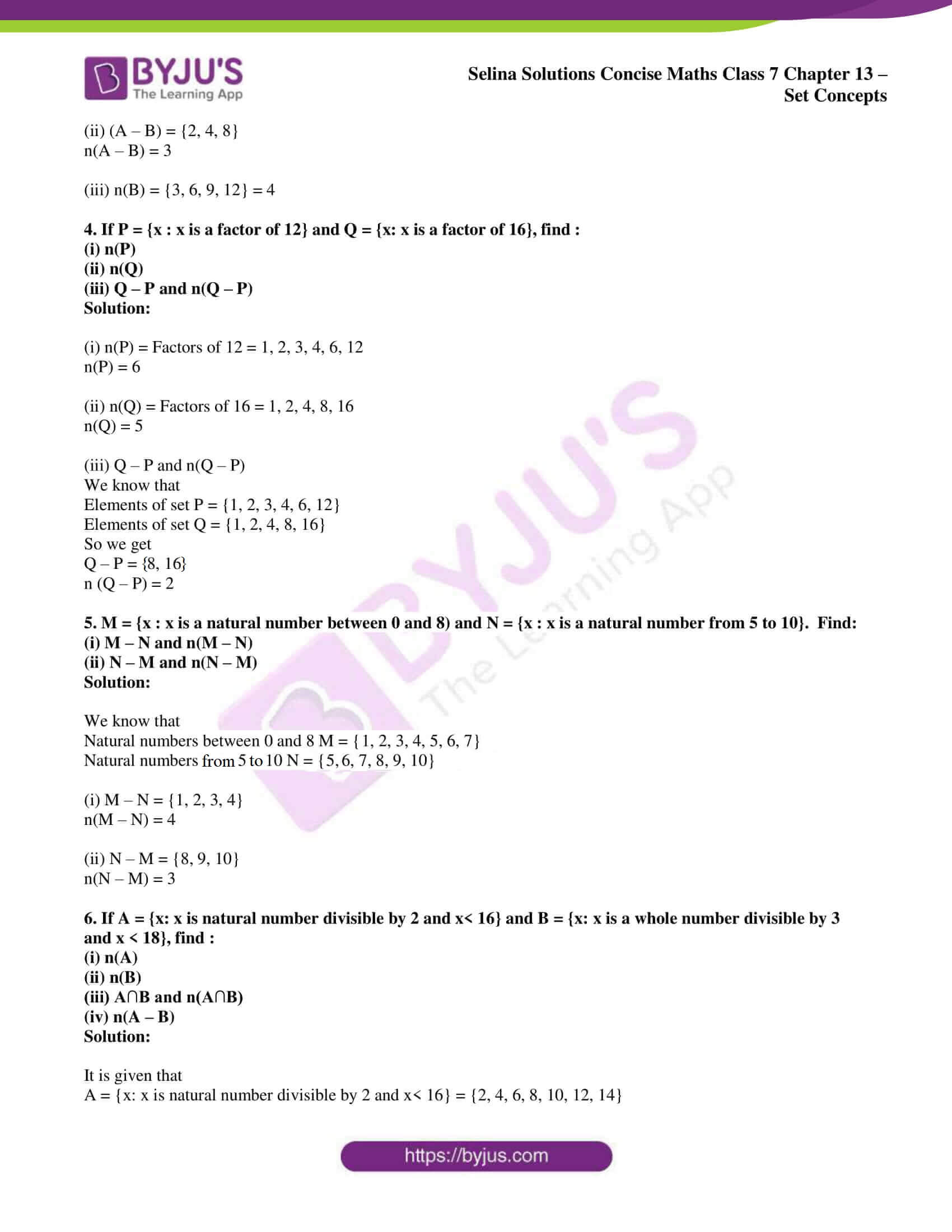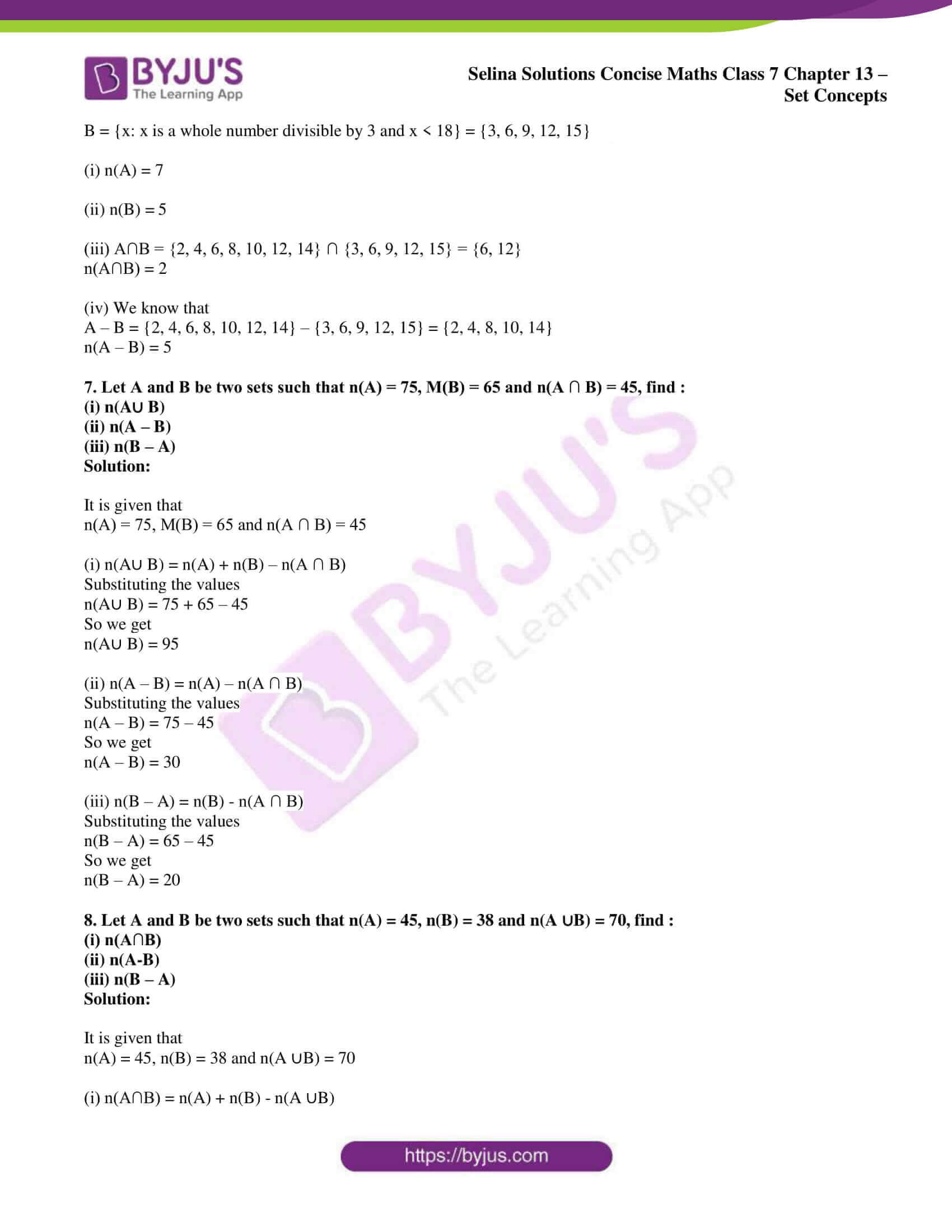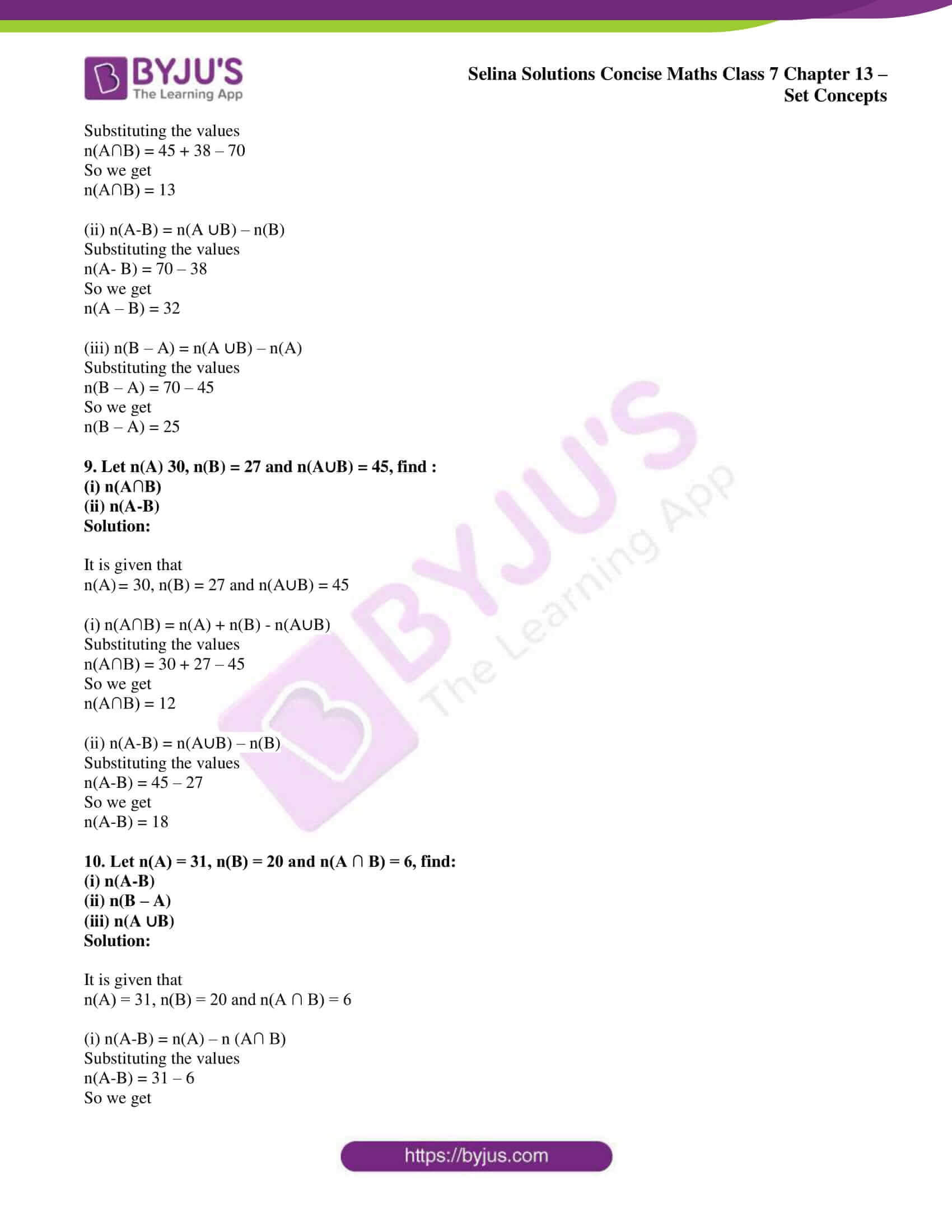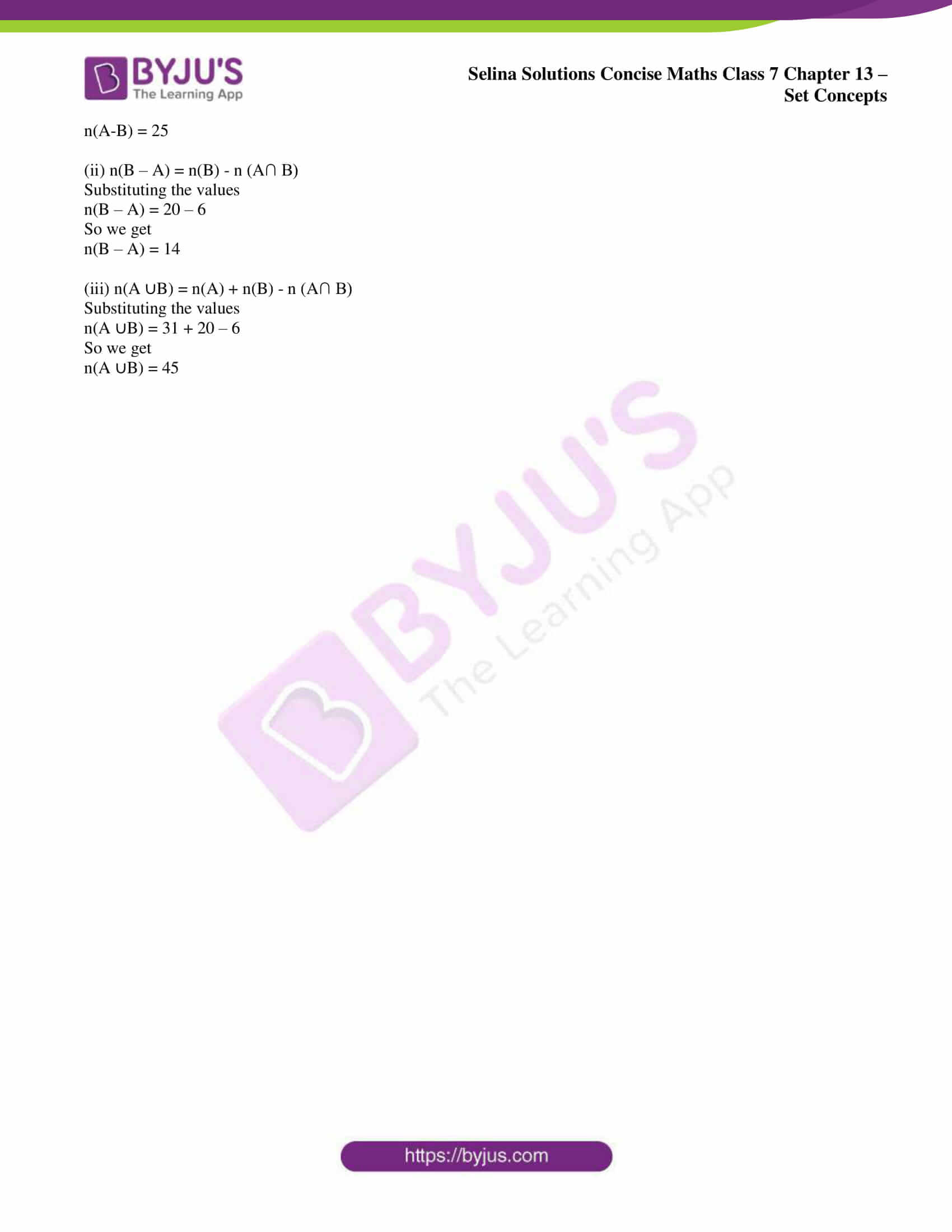### Access other exercises of Selina Solutions Concise Maths Class 7 Chapter 13: Set Concepts

Exercise 13A Solutions

Exercise 13B Solutions

Exercise 13C Solutions

### Access Selina Solutions Concise Maths Class 7 Chapter 13: Set Concepts Exercise 13D

#### Exercise 13D page: 157

1. If A = {4, 5, 6, 7, 8} and B = {6, 8, 10, 12}, find :
(i) A∪B
(ii) A∩B
(iii) A-B
(iv) B-A

Solution:

(i) A∪B

We know that

A∪B = {All the elements from set A and all the elements from set B} = {4, 5, 6, 7, 8, 10, 12}

(ii) A∩B

We know that

A∩B = {Elements which are common to both the sets A and B} = {6, 8}

(iii) A-B

We know that

A – B = {Elements of set A which are not in set B} = {4, 5, 7}

(iv) B-A

We know that

B – A = {Elements of set B which are not in set A} = {10, 12}

2. If A = {3, 5, 7, 9, 11} and B = {4, 7, 10}, find:
(i) n(A)
(ii) n(B)
(iii) A∪B and n(A∪B)
(iv) A∩B and n(A∩B)

Solution:

(i) n(A) = {3, 5, 7, 9, 11} = 5

(ii) n(B) = {4, 7, 10} = 3

(iii) A∪B = {3, 4, 5, 7, 9, 10, 11}

n(A∪B) = 7

(iv) A∩B = {7}

n(A∩B) = 1

3. If A = {2, 4, 6, 8} and B = {3, 6, 9, 12}, find:
(i) (A ∩ B) and n(A ∩ B)
(ii) (A – B) and n(A – B)
(iii) n(B)

Solution:

(i) (A ∩ B) = {6}

n(A ∩ B) = 1

(ii) (A – B) = {2, 4, 8}

n(A – B) = 3

(iii) n(B) = {3, 6, 9, 12} = 4

4. If P = {x : x is a factor of 12} and Q = {x: x is a factor of 16}, find :
(i) n(P)
(ii) n(Q)
(iii) Q – P and n(Q – P)

Solution:

(i) n(P) = Factors of 12 = 1, 2, 3, 4, 6, 12

n(P) = 6

(ii) n(Q) = Factors of 16 = 1, 2, 4, 8, 16

n(Q) = 5

(iii) Q – P and n(Q – P)

We know that

Elements of set P = {1, 2, 3, 4, 6, 12}

Elements of set Q = {1, 2, 4, 8, 16}

So we get

Q – P = {8, 16}

n (Q – P) = 2

5. M = {x : x is a natural number between 0 and 8) and N = {x : x is a natural number from 5 to 10}. Find:
(i) M – N and n(M – N)
(ii) N – M and n(N – M)

Solution:

We know that

Natural numbers between 0 and 8 M = {1, 2, 3, 4, 5, 6, 7}

Natural numbers from 5 to 10 N = {5, 6, 7, 8, 9, 10}

(i) M – N = {1, 2, 3, 4}

n(M – N) = 4

(ii) N – M = {8, 9, 10}

n(N – M) = 3

6. If A = {x: x is natural number divisible by 2 and x< 16} and B = {x: x is a whole number divisible by 3 and x < 18}, find :
(i) n(A)
(ii) n(B)
(iii) A∩B and n(A∩B)
(iv) n(A – B)

Solution:

It is given that

A = {x: x is natural number divisible by 2 and x< 16} = {2, 4, 6, 8, 10, 12, 14}

B = {x: x is a whole number divisible by 3 and x < 18} = {3, 6, 9, 12, 15}

(i) n(A) = 7

(ii) n(B) = 5

(iii) A∩B = {2, 4, 6, 8, 10, 12, 14} ∩ {3, 6, 9, 12, 15} = {6, 12}

n(A∩B) = 2

(iv) We know that

A – B = {2, 4, 6, 8, 10, 12, 14} – {3, 6, 9, 12, 15} = {2, 4, 8, 10, 14}

n(A – B) = 5

7. Let A and B be two sets such that n(A) = 75, M(B) = 65 and n(A ∩ B) = 45, find :
(i) n(A∪ B)
(ii) n(A – B)
(iii) n(B – A)

Solution:

It is given that

n(A) = 75, M(B) = 65 and n(A ∩ B) = 45

(i) n(A∪ B) = n(A) + n(B) – n(A ∩ B)

Substituting the values

n(A∪ B) = 75 + 65 – 45

So we get

n(A∪ B) = 95

(ii) n(A – B) = n(A) – n(A ∩ B)

Substituting the values

n(A – B) = 75 – 45

So we get

n(A – B) = 30

(iii) n(B – A) = n(B) – n(A ∩ B)

Substituting the values

n(B – A) = 65 – 45

So we get

n(B – A) = 20

8. Let A and B be two sets such that n(A) = 45, n(B) = 38 and n(A ∪B) = 70, find :
(i) n(A∩B)
(ii) n(A-B)
(iii) n(B – A)

Solution:

It is given that

n(A) = 45, n(B) = 38 and n(A ∪B) = 70

(i) n(A∩B) = n(A) + n(B) – n(A ∪B)

Substituting the values

n(A∩B) = 45 + 38 – 70

So we get

n(A∩B) = 13

(ii) n(A-B) = n(A ∪B) – n(B)

Substituting the values

n(A- B) = 70 – 38

So we get

n(A – B) = 32

(iii) n(B – A) = n(A ∪B) – n(A)

Substituting the values

n(B – A) = 70 – 45

So we get

n(B – A) = 25

9. Let n(A) 30, n(B) = 27 and n(A∪B) = 45, find :
(i) n(A∩B)
(ii) n(A-B)

Solution:

It is given that

n(A) = 30, n(B) = 27 and n(A∪B) = 45

(i) n(A∩B) = n(A) + n(B) – n(A∪B)

Substituting the values

n(A∩B) = 30 + 27 – 45

So we get

n(A∩B) = 12

(ii) n(A-B) = n(A∪B) – n(B)

Substituting the values

n(A-B) = 45 – 27

So we get

n(A-B) = 18

10. Let n(A) = 31, n(B) = 20 and n(A ∩ B) = 6, find:
(i) n(A-B)
(ii) n(B – A)
(iii) n(A ∪B)

Solution:

It is given that

n(A) = 31, n(B) = 20 and n(A ∩ B) = 6

(i) n(A-B) = n(A) – n (A∩ B)

Substituting the values

n(A-B) = 31 – 6

So we get

n(A-B) = 25

(ii) n(B – A) = n(B) – n (A∩ B)

Substituting the values

n(B – A) = 20 – 6

So we get

n(B – A) = 14

(iii) n(A ∪B) = n(A) + n(B) – n (A∩ B)

Substituting the values

n(A ∪B) = 31 + 20 – 6

So we get

n(A ∪B) = 45